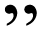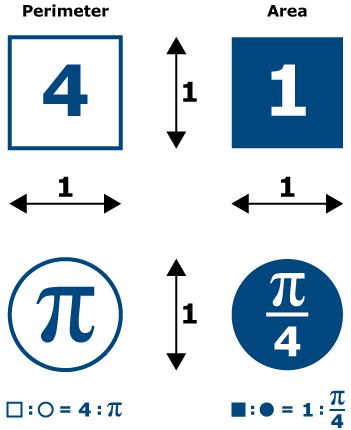## Why Pi is Wrong

Mark Jason Dominus recently blogged on the subject of Why Pi is 3.Simon [Cozens] also asked me why the number came out to be around 3, rather than around 5 or 57, and there I was on much shakier ground. I did not have any clever insights, and all I could do was itemize a bunch of stuff that seemed to bear on the issue. -- Mark Jason DominusMark makes some interesting observations as to why Pi should be 3 (or thereabouts), but doesn't go as far as to question the value of the so-called "fundamental constant" in the first place. Bob Palais, on the other hand, wastes no time in pointing the finger at the "current imposter" in an article written for the Mathematical Intelligencer entitled Pi is Wrong.I know it will be called blasphemy by some, but I believe that π is wrong. [...] The proper value. which does deserve all of the reverence and adulation bestowed upon the current imposter, is the number now unfortunately known as 2π -- Bob PalaisI think Bob's onto something here, but I don't agree with his choice of number for the "new Pi". In fact, I think he's out by a factor of 8. Rather than being twice the currently accepted value for π, I believe the fundamental constant that most intimately represents the geometry of the circle is π/4, or in decimal notation, approximately 0.785.

Consider the diagram below which shows a unit square and a unit circle circle fitting exactly inside it (thanks to norek for pointing out that "In mathematics, a unit circle is a circle with a unit radius, i.e., a circle whose radius is 1."). It doesn't matter what the units are: metres, miles, light years or angstroms will all work equally well. The important thing is that the square has sides of length 1 unit, and the circle a diameter of 1 unit.In the left column we show the perimeter length (the blue outline). For the square it is 4 (sides) x 1 (unit length) = 4 units. For the circle it is given by the equation π x diameter, which in this case is π x 1 = π.

In the right column, we show the area (shaded blue). For the square it is simply 1 x 1 = 1 square unit. For the circle, the area is given by the equation π x radius2. However, we must be careful because we're now talking about the radius (1/2) rather than the diameter (1). So we have π x 1/2 x 1/2 = π x 1/4 = π/4 square units

So square is to circle as 4 is to π, or as 1 is to π/4. The fact that π comes out as a little more than 3 is not particularly remarkable until you realise that it really represents a little more than three quarters rather than a little more than three units.

For a real world example, that means you need π/4 (~0.785) as much cake mix for a round cake tin as you do for a square tin of the same size. In practice "a little more than three-quarters" or "a bit less than four-fifths" are sufficient approximations.

An even closer approximation is given by the infinite series: π/4 ~= 1/1 - 1/3 + 1/5 - 1/7 + 1/9 - 1/11 + ... You can think of this in terms of starting with a square cake and cutting the corners off to make it round. If you're wondering how much of the cake you'll cut off, the answer is a little less than a third. How much less than a third? A little more than a fifth. How much more than a fifth? A little less than a seventh. And so on.

## Summary

Given how deeply π is entrenched in so many areas of mathematics, it's rather unlikely that we're ever going to change its value any time soon, even if we could agree on what the right value should be. π is 3.14... and so shall ever be.

So I'm not really suggesting that Pi is wrong, or needs to be changed, despite the somewhat provocative title of this article (in fact, if anything is "wrong", it's that we traditionally compute the area of a square in terms of the overall width, but use the radius for a circle rather than the diameter). But if you're looking for insight into the mysteries of π and the intimate relation that it has with circles, then π/4 is a good place to start.